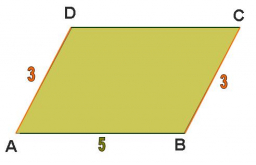# Parallelogram 65954

In the parallelogram ABCD AB = 8, BC = 5, BD = 7. Calculate the magnitude of the angle α = ∠DAB (in degrees).

α =  60 °

### Step-by-step explanation:Did you find an error or inaccuracy? Feel free to write us. Thank you!

Tips for related online calculators
Need help calculating sum, simplifying, or multiplying fractions? Try our fraction calculator.
Cosine rule uses trigonometric SAS triangle calculator.